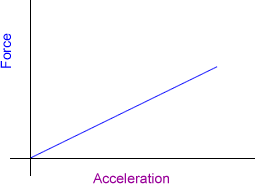# Only the second sum plzz 2) Force applied on a body directly is proportional to acceleration produced in the body. Write an equation to express the situation and plot the graph of the equation taking the constant to be 5 units.Regards

• 0
What are you looking for?